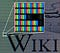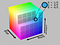# How does a computer see the world?

Computer see the world very differently than us. When we see an image we see people, objects, locations and many more! On the other hand, computers see a 2-dimensional matrix of numbers. However, we have recently started to see that through the advances in embedded vision technology and parallel computing capabilities, computers can now understand on what they see. But exactly how can they do that?

The first step towards this process, and the topic of this article, is to capture the visible light and store it in a way that a computer can manipulate. This happens through electronic devices such as photodiodes, charge-couple devices, and resistors which transform electromagnetic radiation into a 2-dimensional array made of picture elements (pixels) that we refer to as a digital image. Digital images are obtained by sampling and quantization of analog images, this process is called digitization. Sampling takes place in space; equally spaced samples are taken in both horizontal and vertical coordinates. After a sample has been taken quantization is required in order to turn the continuous intensity levels into discrete intensity levels. This can be done by a mapping process that maps continuous spaces into one discrete value. After quantization the discrete value is stored at a position in the array. After the sampling and quantization of the whole analog image the process of generating a digital image is complete. The sampling rate and number of bits used to store the data determines the quality of the digital image .Figure 1: Example of Digitized Image

A digital image can be considered as a two dimensional array I[x, y] of N finite rows and M finite columns, where x and y are spatial coordinates and I[x, y] is called the pixel intensity of the image at that point. A pixel is comprised of three color producing elements each representing one of the three primary colors red, green and blue (Figure 1). This representation is called the RGB model (Figure 2). These primary colors combine together and their variable combinations create the colors that the human eye can see. Each primary color takes values in the space [0, L-1], where L is the number of intensity values. The number of intensity values L is in the form 2k with k denoting the number of bits needed to represent the intensity values. If k is 8 and L is 256 this means that there are 256 possible intensity levels and 8 bits are required to represent all of them. The number of bits needed to store an image is given by M x N x k for grayscale images and by M x N x k x 3 for color images. A color image consists of three component images, one for each of the primary colors. These three images combine on the phosphor screen to produce a composite color image. The number of bits used to represent each pixel in the RGB model is called the pixel depth. The standard used is a 24-bit representation for color images, 8-bits used for each primary color. When all three components are 255, then the resulting color is white. When all three components are 0, the resulting color is black . Grayscale images lack any color information and instead show the mean intensity value of a pixel at a given location. Hence, they do not require three composite images as the intensity values of red, green and blue are all equal for grayscale images. Hence grayscale images require only a third of memory compared to color images because only 8-bits are used to represent a grayscale image . Because of the reduced memory requirements and the fact that they still hold valuable object and scene information Grayscale images are the standard for applications such as object detection motion detection and 3D scene reconstruction.Figure 2: The RGB Model

There are different ways that a computer can manipulate a digital image and can be divided into three categories according with the objective of each one  . First there is the category of image processing that involves tasks such as image enhancement and noise removal. In image processing an image is processed and the result of this processing is another image that is improved according to what the goal is. Next, there is image analysis. Again the input is an image and the results are measurements that give some statistical analysis of the image. Finally there is the category of computer vision and image understanding. Tasks in this category include object matching and recognition. The goal here is, given an image to extract a high level description of the image.

REFERENCES

1. Rafael C. Gonzalez, Richard E. Woods, Steven L. Eddins , “Digital Image Processing”, (Upper Saddle River NJ: Prentice Hall), Chapter 2, P1–31.
2. Rafael C. Gonzalez, Richard E. Woods , “Digital Image Processing”, second edition, (Upper Saddle River NJ: Prentice Hall, 2002), Chapter 6, P34–71.
3. Christos Kyrkou, “Neural-Network-Based Face Detector Implementation on a Virtex II PRO FPGA Platform”, BSc Thesis, University of Cyprus, Nicosia, June 2008.

PhD in Computer Engineering, Self-Driving Car Engineering Nanodegree, Computer Vision, Visual Perception and Computing

## More from Christos Kyrkou

PhD in Computer Engineering, Self-Driving Car Engineering Nanodegree, Computer Vision, Visual Perception and Computing